# GMAT Math : DSQ: Calculating the length of the side of a quadrilateral

## Example Questions

### Example Question #1 : Other Quadrilaterals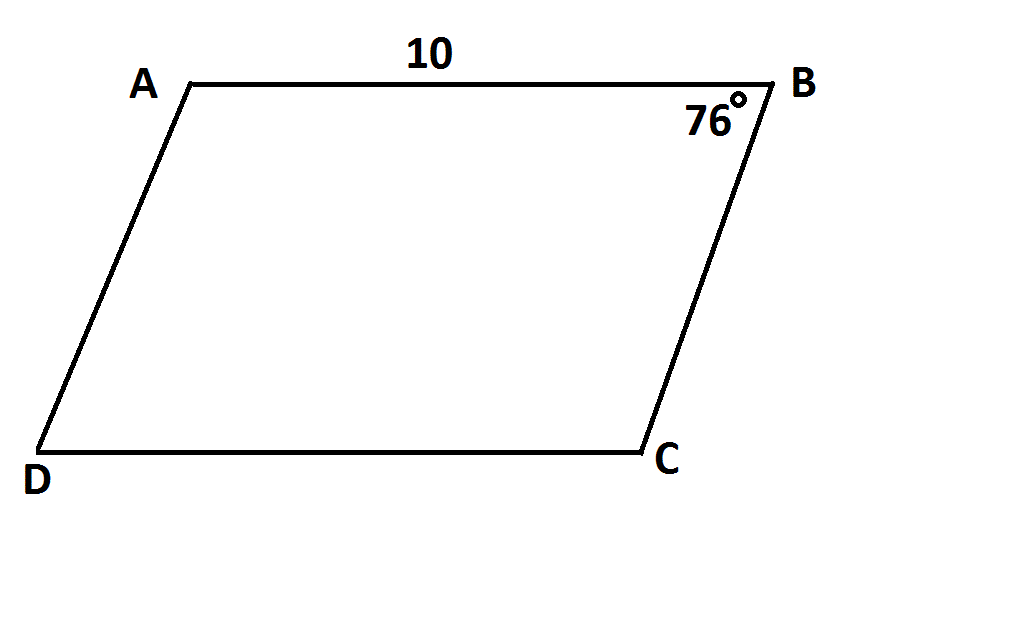NOTE: Figure NOT drawn to scale.

Is the above figure a parallelogram?

Statement 1: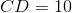Statement 2: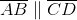Statement 1 ALONE is sufficient to answer the question, but Statement 2 ALONE is NOT sufficient to answer the question.

BOTH statements TOGETHER are insufficient to answer the question.

EITHER statement ALONE is sufficient to answer the question.

Statement 2 ALONE is sufficient to answer the question, but Statement 1 ALONE is NOT sufficient to answer the question.

BOTH statements TOGETHER are sufficient to answer the question, but NEITHER statement ALONE is sufficient to answer the question.

BOTH statements TOGETHER are sufficient to answer the question, but NEITHER statement ALONE is sufficient to answer the question.

Explanation:

Knowing one pair of sides of a quadrilateral to be congruent is not alone sufficient to prove that figure to be a parallelogram, nor is knowing one pair of sides of a quadrilateral to be parallel. But knowing both about the same pair of sides is sufficient by a theorem of parallelograms.

### Example Question #2 : Other Quadrilaterals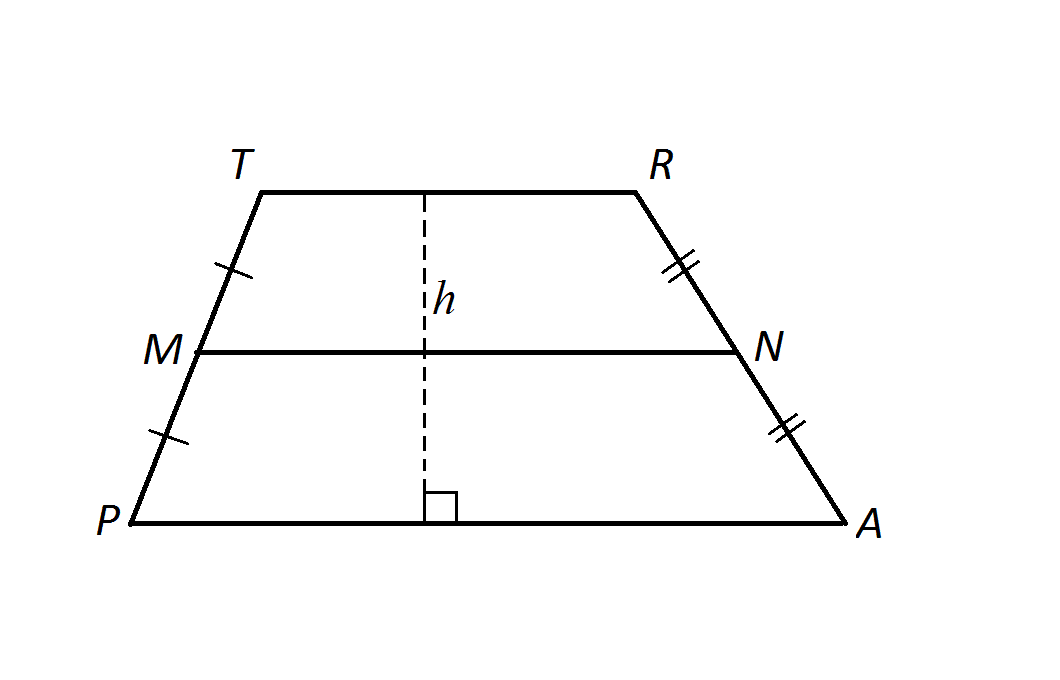Notes:refers to the length of the entire dashed line. Figure not drawn to scale.

Calculate, the height of the large trapezoid.

Statement 1: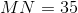Statement 2: The area of the trapezoid is 7,000.

Statement 1 ALONE is sufficient to answer the question, but Statement 2 ALONE is NOT sufficient to answer the question.

EITHER statement ALONE is sufficient to answer the question.

BOTH statements TOGETHER are insufficient to answer the question.

Statement 2 ALONE is sufficient to answer the question, but Statement 1 ALONE is NOT sufficient to answer the question.

BOTH statements TOGETHER are sufficient to answer the question, but NEITHER statement ALONE is sufficient to answer the question.

BOTH statements TOGETHER are sufficient to answer the question, but NEITHER statement ALONE is sufficient to answer the question.

Explanation:

Consider the area formula for a trapezoid: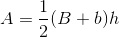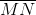is the midsegment of the trapezoid, which, by the Trapezoid Midsegment Theorem, has length equal to the mean of the bases - in other words,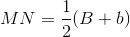. The area formula can be expressed, after substitution, as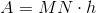So, if you know both the area and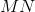- but not just one - you can find the height by dividing.

### Example Question #3 : Other Quadrilaterals

Consider parallelogram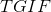.

I) The perimeter ofis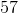light years.

II) Side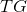islight years and is equivalent to side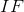.

Find the length of side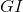.

Both statements are needed to answer the question.

Statement II is sufficient to answer the question, but statement I is not sufficient to answer the question.

Either statement is sufficient to answer the question.

Statement I is sufficient to answer the question, but statement II is not sufficient to answer the question.

Both statements are needed to answer the question.

Explanation:

We can work backwards from the perimeter to find the length of the unknown side.

I) Gives us the perimeter.

II) Gives us two of the sides.

In a parallelogram there are two sets of corresponding sides. We can use I) and II) to write the following equaiton, where l is the length of our wanted side.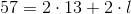Solve for l to find our final side: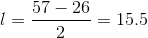### Example Question #4 : Other Quadrilaterals

Calculate the side of a square.

Statement 1:  A circle with an area ofis enclosed inside the square and touches all four edges of the square.

Statement 2:  A circle with a circumference ofencloses the square and touches all four corners of the square.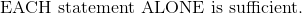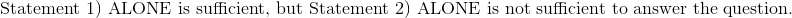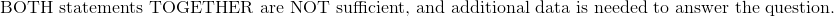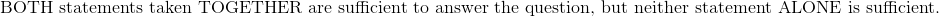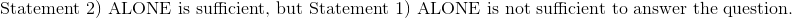Explanation:

Statement 1:  A circle with an area ofis enclosed inside the square and touches all four edges of the square.

Write the formula for the area of the circle.After substituting the value of the area and solve for radius, the radius is 1.  The side length of the square is double the length of the radius.

Statement 2:  A circle with a circumference ofencloses the square and touches all four corners of the square.

Write the formula for the circumference of the circle.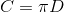After substituting the value of the circumference and solve for diameter, the diameter of the circle is 1.  This diameter represents the diagonal of the square.  Because the square length and width are equal dimensions, it is possible to solve for the lengths of the square by Pythagorean Theorem knowing just the length of the square diagonal.

Therefore:### Example Question #5 : Other Quadrilaterals

Consider isosceles trapezoid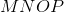.

I)has a perimeter of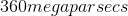.

II) The larger base ofis 45 times bigger than the smaller base.

Find the length of the two legs of.

Statement I is sufficient to answer the question, but Statement II is not sufficient to answer the question.

Either statement is sufficient to answer the question.

Statement II is sufficient to answer the question, but Statement I is not sufficient to answer the question.

Both statements are needed to answer the question.

Explanation:

Consider isosceles trapezoid.

I)has a perimeter of.

II) The larger base ofis 45 times bigger than the smaller base.

Find the length of the two legs of.

To find the length of the legs of a trapezoid, we need the perimeter and the two bases. Then, we can subtract the sum of the two bases from the perimeter and divide by two to find the lengths of the sides we are looking for.

Statement I gives us the perimeter of.

Statement II relates the two bases of.

We are told thatis an isosceles trapezoid, which means its two legs are equal; however, we still have too many unknowns and not enough equations. There is no way to solve this without more information.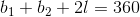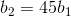We still have three unknowns and two equations, so we cannot solve this system of equations.

Tired of practice problems?

Try live online GMAT prep today.